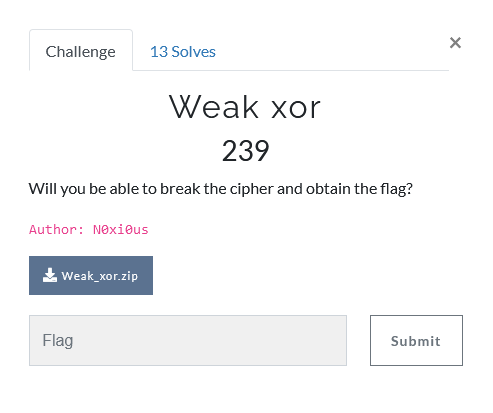# NetOn CTF 2021 - Weak xor

Cryptography – 239 pts (13 solves) – Chall author: N0xi0us

XOR with known plaintext allows us to easily recover the key.

## ChallengeThe challenge provides us with an XOR encoded flag in hex, and the code they used to encode it

#!/usr/bin/python3
import os
flag = open('flag.txt', 'r').read().strip().encode()
key = os.urandom(6)
xored = b''
for i in range(len(flag)):
xored += bytes([flag[i] ^ key[i % len(key)]])
print(f"Flag : {xored.hex()}")


## Solution

So they used 6 random bytes as the XOR key… Well, time to brute-force our way to this key. 6 bytes is an incredibly short key length and can be cracked in no time. I made a simple Python script to do this.

#!/usr/bin/env python3

# Encrypted flag
chex = '5bbed19a19234dcbf78a3e0b4abcb5e5330721a4b5a3322a7397b5a22a'
cbyt = cint.to_bytes(29,'big')
# Example flag
tag = b'NETON{'
# Final key
key = b''

# We know the length of the key is 6 bytes (!)
while len(key) < 6:
# Loop over possible bytes
for i in range(256):
# Get the byte
if i < 16:
tryhex = bytes.fromhex('0'+hex(i)[2:])
else:
tryhex = bytes.fromhex(hex(i)[2:])
# Create trial key
trykey = key + tryhex
# XOR encrypted flag bytes with trial key
xor = b''
for i in range(len(cbyt)):
xor += bytes([cbyt[i] ^ trykey[i % len(trykey)]])
# Check XOR result with example flag
if xor[:len(key)+1] == tag[:len(key)+1]:
key += tryhex
break
# Print results
print(key)
print(xor)


which happily returns us our desired key and flag : )

b'\x15\xfb\x85\xd5WX'
b'NETON{X0r_iS_G00d_4_0verfl0w}'


Note that the key can also be directly retrieved from the ciphertext as the first 6 plaintext bytes are known, I was just a lil’ script kiddie…